# Microelectronics

## 04 Inverter

### Prof. Dr. J—rg Vollrath

03 MOSFET## Video of lecture 04 14.4.2021

 Video is not visible, most likely your browser does not support HTML5 video Lðnge: 01:25:00 0:2:21 1um PFET 40 uA/V-2 0:5:30 RC Delay 0:7:0 Inverter 0:8:30 Transfer curve 0:15:48 Curve shift with width of NFET 0:23:23 Added of C 0:27:13 Switching of inverter 0:32:53 Add load and driver for delay measurement 0:41:13 Attach probe with R and C 0:42:50 Layout of standard cells and naming 0:51:33 List of rules and guidelines 0:52:16 Standard cell good example 0:55:23 Pass gate and transmission gate 0:58:16 NFET pass gate maximum voltage 1:1:3 Transfer gate 1:7:9 Pass gate simulation 1:12:53 Power consumption 1:17:43 Area per transistor

# Overview

• Technologies: ASIC, FPGA, Microcontroller
• You have a basic understanding of a MOSFET
• Gate, source, drain, bulk/well: VGS, VDS, VBS
• Threshold voltage Vth, drain source current IDS
• Transfer and output IV curve
• Output resistance RDSon
• Layout: Width (W), length (L)
• Capacitance and RDSon, C delay
• Inverter
• IV curve
• Propagation delay
• Standard cells

# Inverter schematic and layout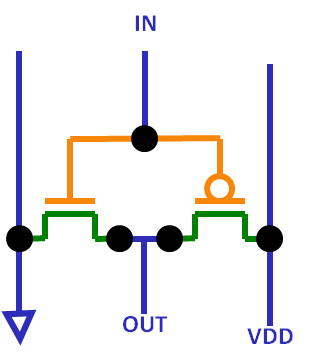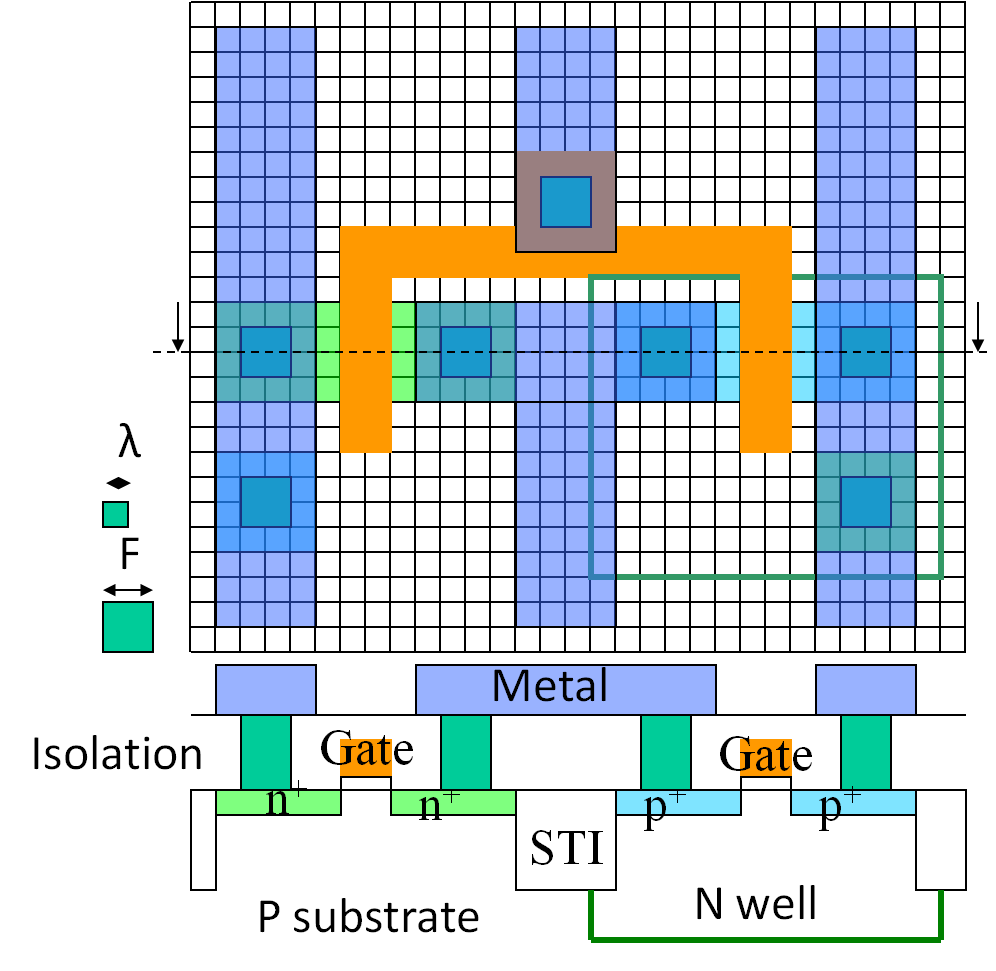Schematic and layout are representing an inverter.
An inverter uses a NMOSFET and a PMOSFET.
The schematic shows the components and connections.
The layout has added different colors for different layers of the manufacturing process and each component.
These colors are transferred into the schematic showing the relationship between layout and schematic.
Wiring and transistor gates and diffusion have a minimum physical size of width and length of F.
There are also minimum distances required to prevent shorts.

It is difficult to automatically transform one view into the other.
A schematic is drawn to be able to easily understand the circuit. On the left is GND, on the right VDD, on the top inputs, on the bottom outputs.
In a schematic connection between 2 pins can be accomplished by labeling the pins with the same names. Lines can cross without resulting in short circuits.
A layout is drawn for symmetric and regular patterns, avoiding signal cross coupling and shape distortion, and having minimum size.

# Operation of the MOSFET switchNMOS Logic 1 turns the switch on Logic 0 turns the switch off PMOS Logic 0 turns the switch on Logic 1 turns the switch off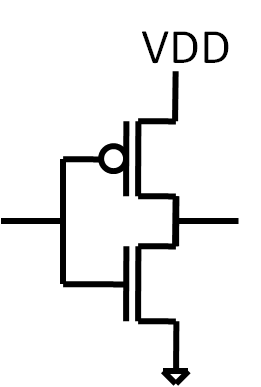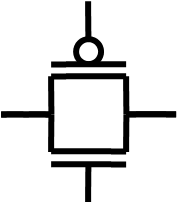Inverter Transmission gate

# MOSFET switch model

 Switch model RDSon: resistance Cox: capacitance RC delay $t_{pLH} = 0.7 \cdot R_{DSPon} \cdot C$ $t_{pHL} = 0.7 \cdot R_{DSNon} \cdot C$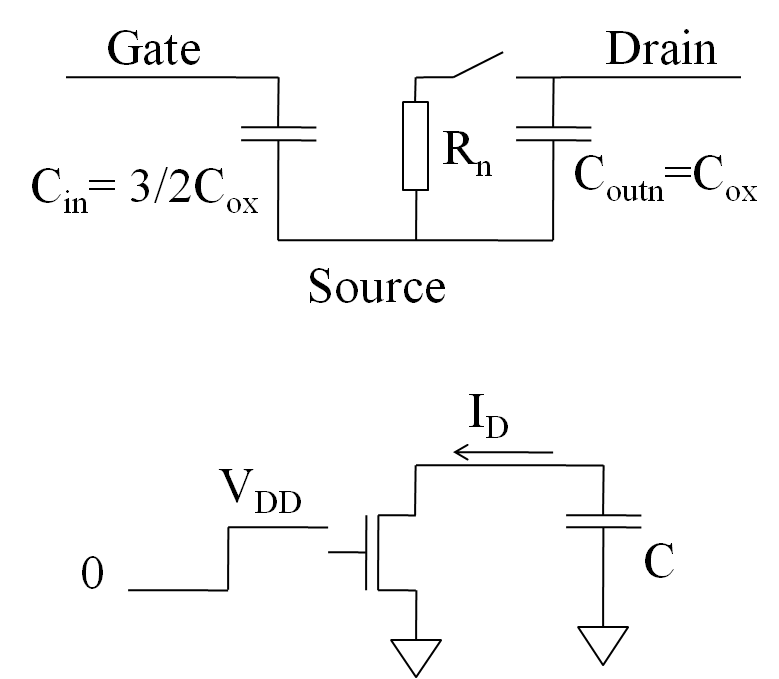The equivalent input capacitance for a CMOS inverter can be calculated.
The input goes from low (GND) to high (VDD).
The output goes from high (VDD) to low (GND).
1/2 Cox is between Gate and source and 1/2 Cox is between gate and drain.
The gate drain capacitance sees twice the voltage swing giving an equivalent capacitance of Cox. Therefore the total input capacitance is:
$C_{in} = \frac{3}{2} C_{ox}$
The total output capacitance is:
$C_{out} = C_{ox}$
An amplifier with a high voltage gain of AV has an input capacitance of:
$C_{in} = (A_{V} + 2) \frac{1}{2} C_{ox}$
This is called the Miller effect.

The propagation delay tp can be different for rising (LH) or falling (HL) edge at the output due to the on resistance of the PFET or NFET.
To take into account input capacitance and output resistance for a delay measurement a real source is needed before the device under test (DUT) and a real load is needed after the DUT.
Be careful when simulations are done with ideal sources and no load.

# Propagation delay

 Charging of a capacitor (RC Delay)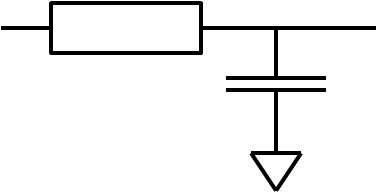Propagation Delay tP: Blue arrow Different between rising and falling edge. Different active transistors.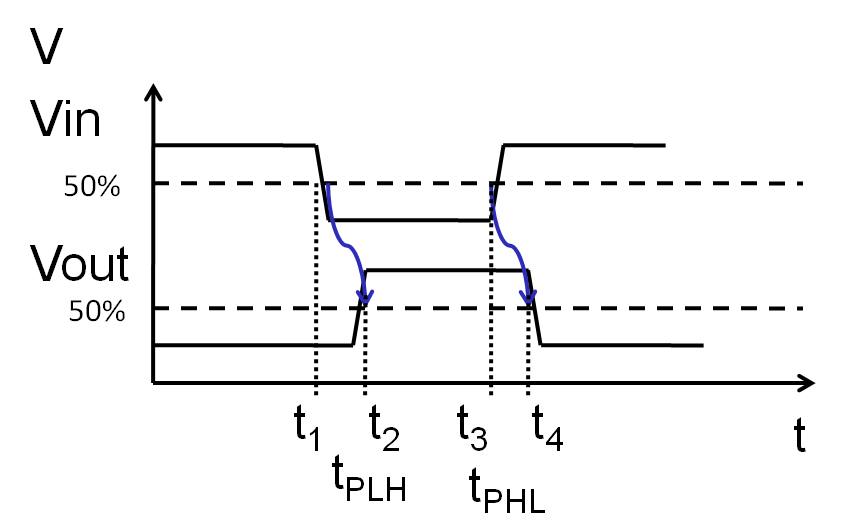# Inverter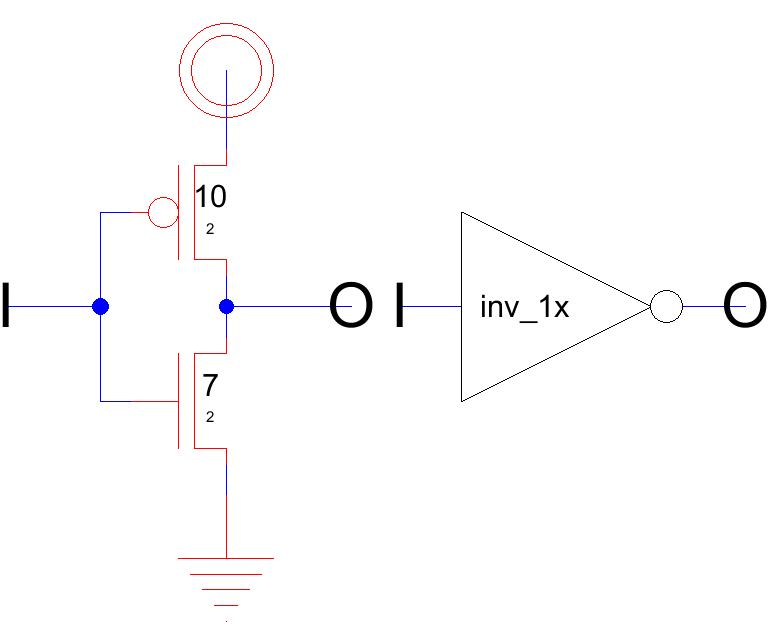A schematic and logic symbol of an inverter is displayed. The PFET at the top and NFET at the bottom can be seen. The length and width are annotated at the transistors. Since drawings are done with a precision of λ = 1/2 F width and length is given in λ. The inverter can also give the sizing of PFET and NFET. Here an inverter with 1x size is given.

# Transfer curve of an inverter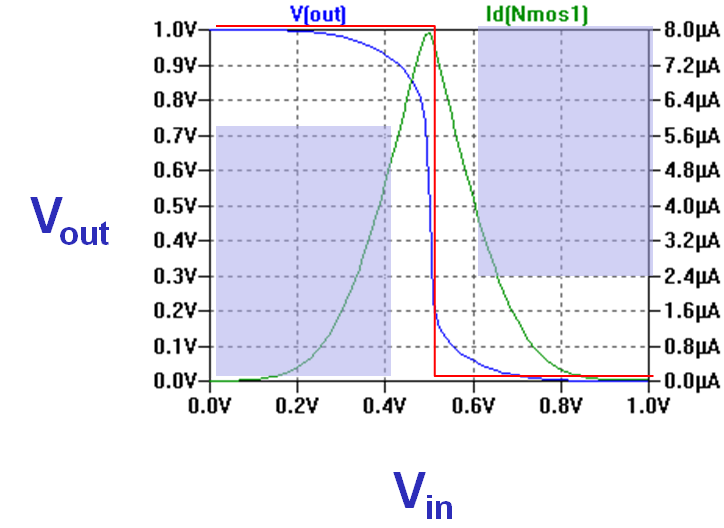# Switching of an inverter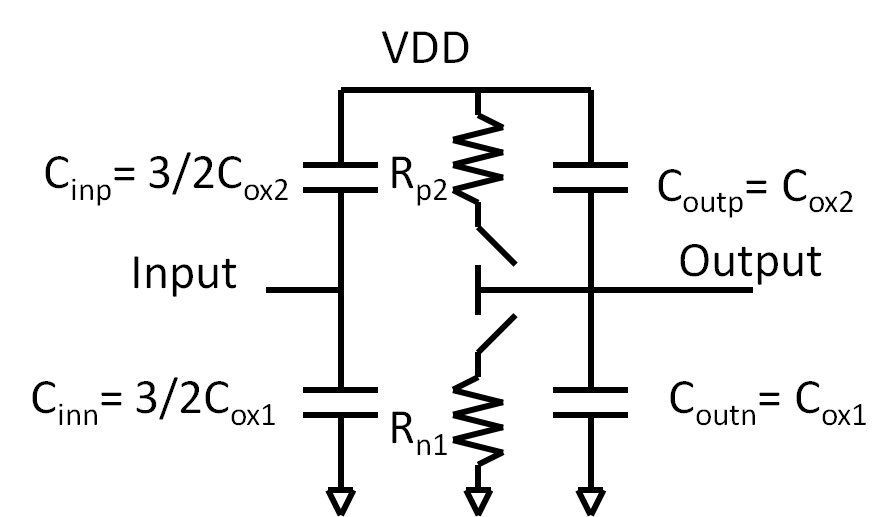# Standard cell: NAND layout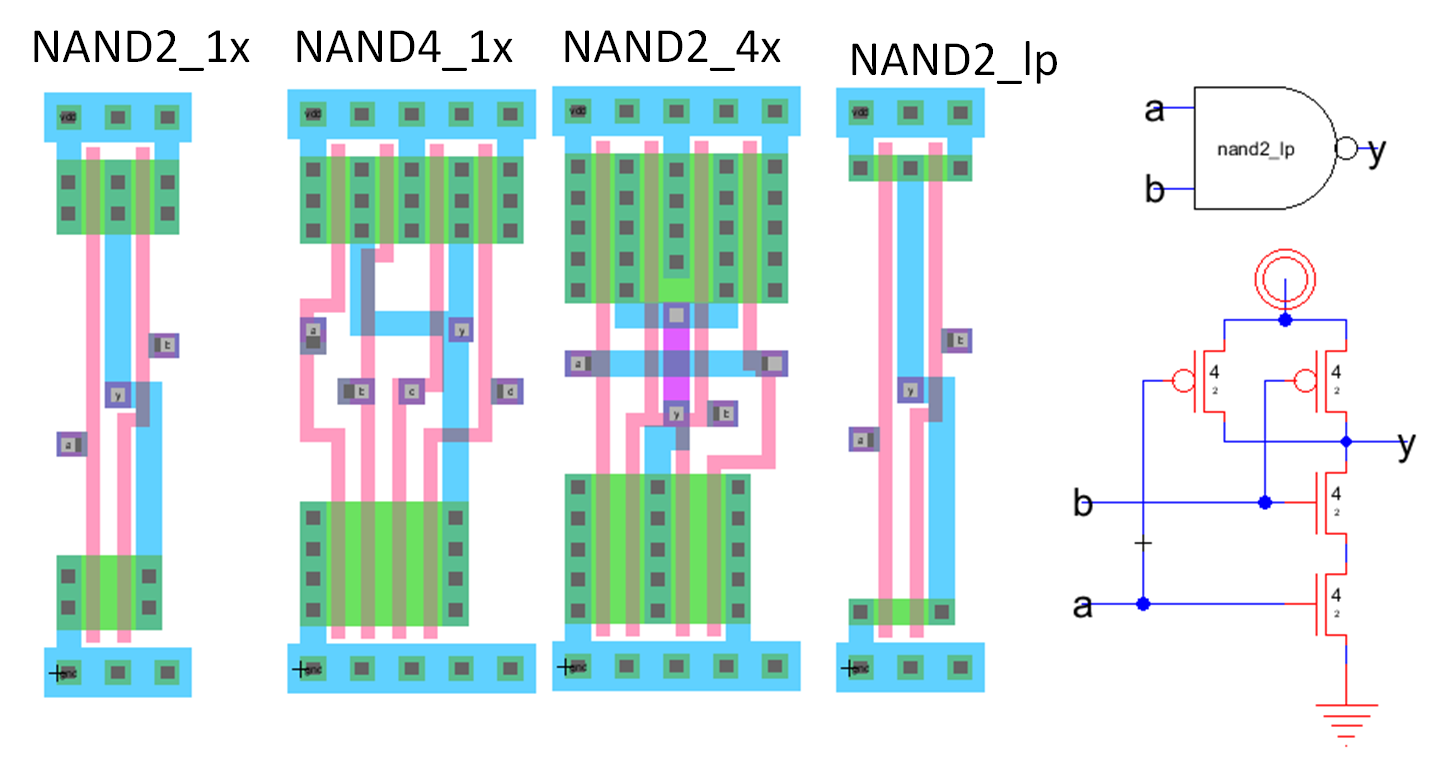Cell Discussion:
Transistors
Width determined by number of input and outputs
Same height, Names, vertical horizontal lines, exports, Hierarchy

# Standard cell: Details

 Start are minimum size transistors for low power, below 50nm only unit transistors are used (FINFET) Horizontal lines: SD-area/diffusion, M1, M3, Vertical lines: polysilicon, M2, M4,.. Lines should have minimum bends Contacts should be placed on a grid Input and outputs should be M2 in the center to make vertical routing possible. Since width of cell is limited by input and output contacts no shared VDD, gnd connections are used. Optimize later, when problems are encountered.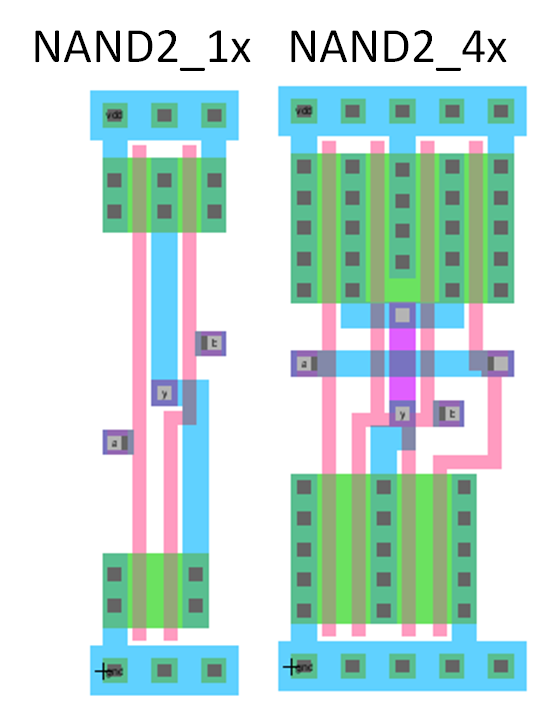# Standard cell sub 50 nm Feature Size

 Start are minimum size transistors for low power, below 50nm only unit transistors are used (FINFET) Horizontal lines: SD-area/diffusion, M1, M3, Vertical lines: polysilicon, M2, M4,.. Lines should have minimum bends Contacts should be placed on a grid Input and outputs should be M2 and in the center have different x position to make vertical routing possible. Since width of cell is limited by input and output contacts no shared VDD, gnd connections are used. Optimize later, when problems are encountered.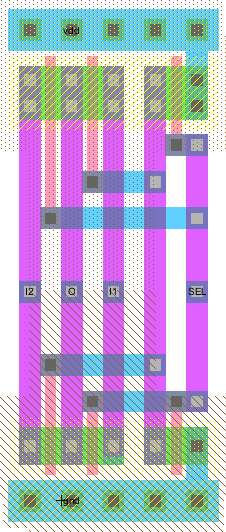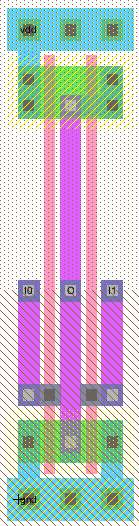# Pass gate and transmission gate

 Pass gate (PG) and transmission gate (TG) Voltage limit: NMOS: Vout = min(Vgs-Vth,Vin), PMOS: Vout = max(Vth, Vin) Multiplexer: MUX, DEMUX Inverter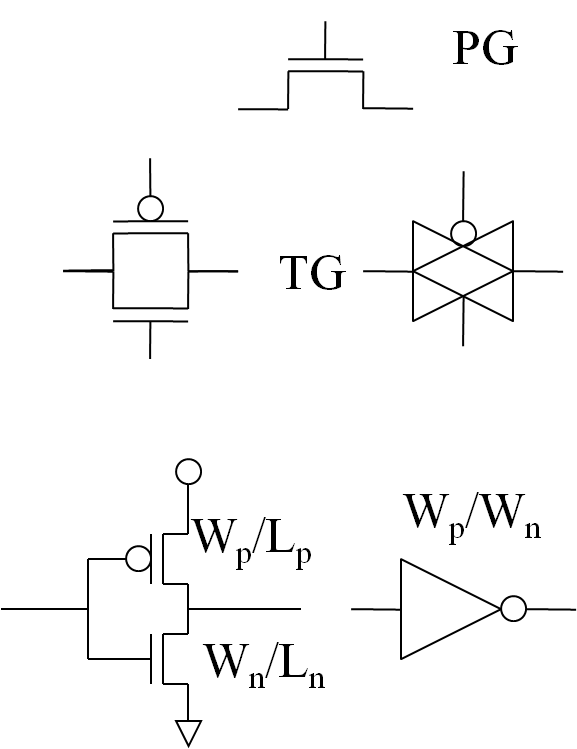# Switching of a pass gate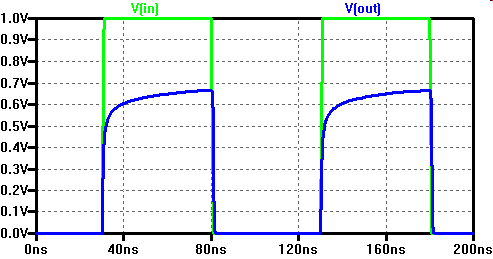Delay through a pass gate:
$t_{pPG} = 0.7 \cdot R_{DSon} \cdot ( C_{L} + \frac{C_{ox}}{2} )$

# Switching of a transmission gate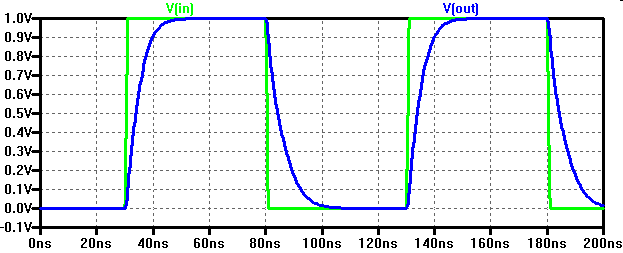Delay through a transmission gate:
$t_{pPG} = 0.7 \cdot (R_{DSonn} || R_{DSonp} ) \cdot ( C_{L} + \frac{C_{oxn}}{2} + \frac{C_{oxp}}{2} )$

# Next:

05 IC Manufacturing process technology